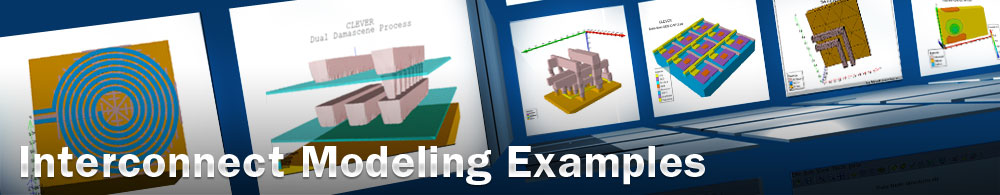3D MIM capacitance

## questex12.in : 3D MIM capacitance

Requires: QUEST Minimum Versions: QUEST 2.0.2.R

This example demonstrates the use of QUEST for 3D MIM capacitance simulation.

Introduction:

The Metal-Insulator-Metal (MIM) capacitor is a key passive component in RF and analog integrated circuits. MIM capacitors have attracted great attention because of their high capacitance density that supplies a small area, increases circuit density and further reduces fabrication cost.

Running the Example

To load and run this example, select the Load example button in DeckBuild. This will copy the input file, GDSII file and results to your current working directory. Select the run button to execute the example.

Results analysis

The objective of the analysis is to extract the R,L and C parameter of the simple RLC MIM device equivalent model.

For that purpose we use the B element of the ABCD matrix (questex12_abcd.dat). From the imaginary part of the B element we extract C and L according to the following equation B_I=Lw -1/CW using the build-in optimizer by the JavaScript capability. The real part of B gives directly the Resistance R.

To run the script use the RUNJAVASCRIPT command or type the following command:

quest -v -b javascript_name.js

This example also shows the DBinternal capability to investigate the MIM Thickness effect on the capacitance value. Please refer to DeckBuild example DOE: Design of Experiment Examples for detailed explanation of the DBinternal syntax.